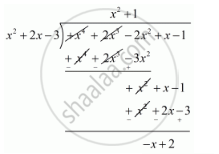Share

# What Must Be Added to the Polynomial F(X) = X4 + 2x3 − 2x2 + X − 1 So that the Resulting Polynomial is Exactly Divisible By X2 + 2x − 3 ? - CBSE Class 10 - Mathematics

ConceptDivision Algorithm for Polynomials

#### Question

What must be added to the polynomial f(x) = x4 + 2x3 − 2x2 + x − 1 so that the resulting polynomial is exactly divisible by x2 + 2x − 3 ?

#### Solution

We know that,

f(x) = g(x) x q(x) + r(x)

f(x) - r(x) = g(x) x q(x)

f(x) + {- r(x)} = g(x) x q(x)

Clearly , Right hand side is divisible by g(x).

Therefore, Left hand side is also divisible by g(x).Thus, if we add - r(x) to f(x), then the resulting polynomial is divisible by g(x).

Let us now find the remainder when f(x) is divided by g(x).Hence, we should add - r(x) = x - 2 to f(x) so that the resulting polynomial is divisible by g(x).

Is there an error in this question or solution?

#### APPEARS IN

RD Sharma Solution for 10 Mathematics (2018 to Current)
Chapter 2: Polynomials
Ex. 2.30 | Q: 7 | Page no. 58

#### Video TutorialsVIEW ALL 

Solution What Must Be Added to the Polynomial F(X) = X4 + 2x3 − 2x2 + X − 1 So that the Resulting Polynomial is Exactly Divisible By X2 + 2x − 3 ? Concept: Division Algorithm for Polynomials.
S